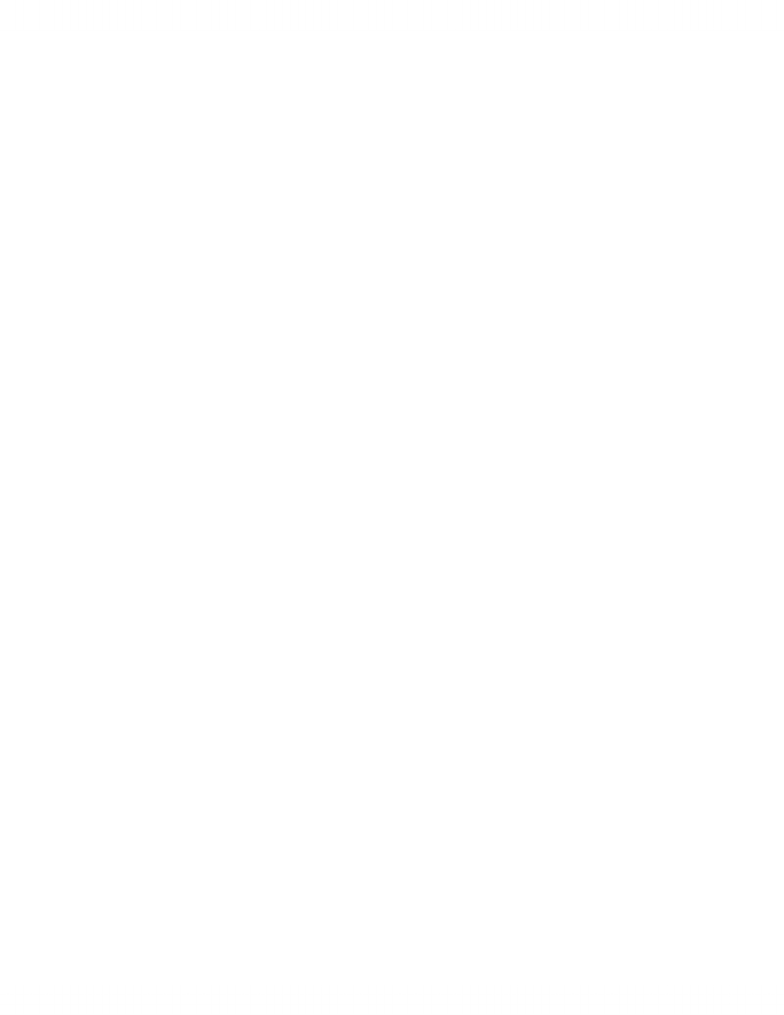Textbook Notes (290,000)
CA (170,000)
UTSG (10,000)
SOC (1,000)
Chapter 14

# summary for chapter 14

Department
Sociology
Course Code
SOC202H1
Professor
Brent Berry
Chapter
14

This preview shows half of the first page. to view the full 3 pages of the document.Chapter 14
The key element of statistical imagination is the analysis if cause and consequences
with the goal to predicting future events
The test estimate of a dependent variable can be imposed if we can identify
independent variable and extend the analysis to the bivariate level
Correlation: is a systematic change in the scores of 2 interval ration variable (when
the change in one variable change in tandem with the measurement of the other)
2 variable correlated if their measurement consistently change together form case to
case
Simple bivariate linear correlation and regression analysis
1)Procedure of improving best estimate of Y by accounting for its relationship with
X (main goal to reduce error in prediction)
2)Central idea: use a straight line to improve best estimate of an interval ratio (y)
for all values of interval ration (x)
3)The formula to estimate Y Y= a+bX (applies only when both variable are
interval ration- used only with linear relationship between X and Y
Scartterplot: a two-dimensional grid of the coordinate of two interval-ration
variables X and Y
Coordinate: a point on a scatterplot where the values of X and Y are plotted for a
case
Linear patterns: on where the coordinate of the scatterplot fall into a shape of a
straight line
The horizontal axis provided values of a variable X (x axis) and the vertical axis
represents value of the second interval variable Y (y axis)
X-> the predicator (independent) y-> the dependent variable
When 2 variables increase in tandem it is called a positive relationship/ when x
increases and y decreases it is a negative correlation
When no correlation exist between x and y, the mean of Y remains the best estimate
of Y for all values of X
www.notesolution.com